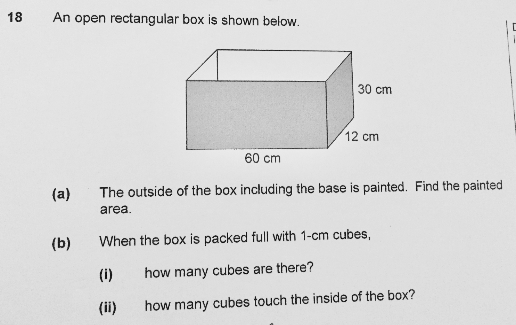# QuestionAnyone help me with attached question

Thanks

Area of front and back → 2 × (60 × 30) = 3600 cm2

Area of 2 sides → 2 × (30 × 12) = 720 cm2

Area of bottom → 60 × 12 = 720 cm2

(a) Total painted area → 3600 + 720 + 720 = 5040 cm2

(b)

(i) Number of cubes to fill the box → 60 × 30 × 12 = 21600

(ii)

Cubes touching front and back → 2 × (60 × 30) = 3600

To avoid double counting, width of box is now (12 – 2) = 10 cm to exclude the cubes touching front and back

Cubes touching the two sides → 2 × (30 × 10) = 600

Again, to avoid double counting, length is now (60 – 2) = 58cm

Cubes touching bottom of box → 58 × 10 = 580

Total number of cubes touching the inside → 3600 + 600 + 580 = 4780

Thanks for explanation

0 Replies 0 Likes

(a)

painted area ——- (60 x 12) + (2 x 60 x 30) + (2 x 12 x 30) = 5040

(b)

(i)

60 x 12 x 30 = 21600

(ii)

21600 –  58 x 10 x 29 = 4780

Ans : (a) 5040 sq cm; (b)(i) 21600 cubes, (ii) 4780 cubes.

0 Replies 2 Likes